# 因子分析——python

1.导入库

2.读取数据

3.充分性检测

3.1 Bartlett's球状检验

3.2 KMO检验

4.选择因子个数

4.1 特征值和特征向量

4.2 可视化展示

4.3 可视化中显示中文不报错

5.因子旋转

5.1 建立因子分析模型

5.2 查看因子方差-get_communalities()

5.3 查看旋转后的特征值

5.4 查看成分矩阵

5.5 查看因子贡献率

6.隐藏变量可视化

7.转成新变量

# 一、起源

因子分析的起源是这样的：1904年英国的一个心理学家发现学生的英语、法语和古典语成绩非常有相关性，他认为这三门课程背后有一个共同的因素驱动，最后将这个因素定义为“语言能力”。

基于这个想法，发现很多相关性很高的因素背后有共同的因子驱动，从而定义了因子分析，这便是因子分析的由来。

# 二、基本思想

我们再通过一个更加实际的例子来理解因子分析的基本思想：

现在假设一个同学的数学、物理、化学、生物都考了满分，那么我们可以认为这个学生的理性思维比较强，在这里理性思维就是我们所说的一个因子。在这个因子的作用下，偏理科的成绩才会那么高。

到底什么是因子分析？就是假设现有全部自变量x的出现是因为某个潜在变量的作用，这个潜在的变量就是我们说的因子。在这个因子的作用下，x能够被观察到。

因子分析就是将存在某些相关性的变量提炼为较少的几个因子，用这几个因子去表示原本的变量，也可以根据因子对变量进行分类

因子分子本质上也是降维的过程，和主成分分析（PCA）算法比较类似。

# 三、算法用途

因子分析法和主成分分析法有很多类似之处。因子分析的主要目的是用来描述隐藏在一组测量到的变量中的一些更基本的，但又无法直接测量到的隐性变量。因子分析法也可以用来综合评价。

其主要思路是利用研究指标的之间存在一定的相关性，从而推想是否存在某些潜在的共性因子，而这些不同的潜在的共性因子不同程度地共同影响着研究指标。因子分析可以在许多变量中找出隐藏的具有代表性的因子，将共同本质的变量归入一个因子，可以减少变量的数目。

# 四、因子分析步骤

• 对所给的数据样本进行标准化处理

• 计算样本的相关矩阵R

• 求相关矩阵R的特征值、特征向量

• 根据系统要求的累积贡献度确定主因子的个数

• 计算因子载荷矩阵A

• 最终确定因子模型

• # 五、factor_analyzer库

利用Python进行因子分析的核心库是：factor_analyzer

``````pip install factor_analyzer
``````

这个库主要有两个主要的模块需要学习：

• factor_analyzer.analyze（重点）

• factor_analyzer.factor_analyzer

• # 四、实例详解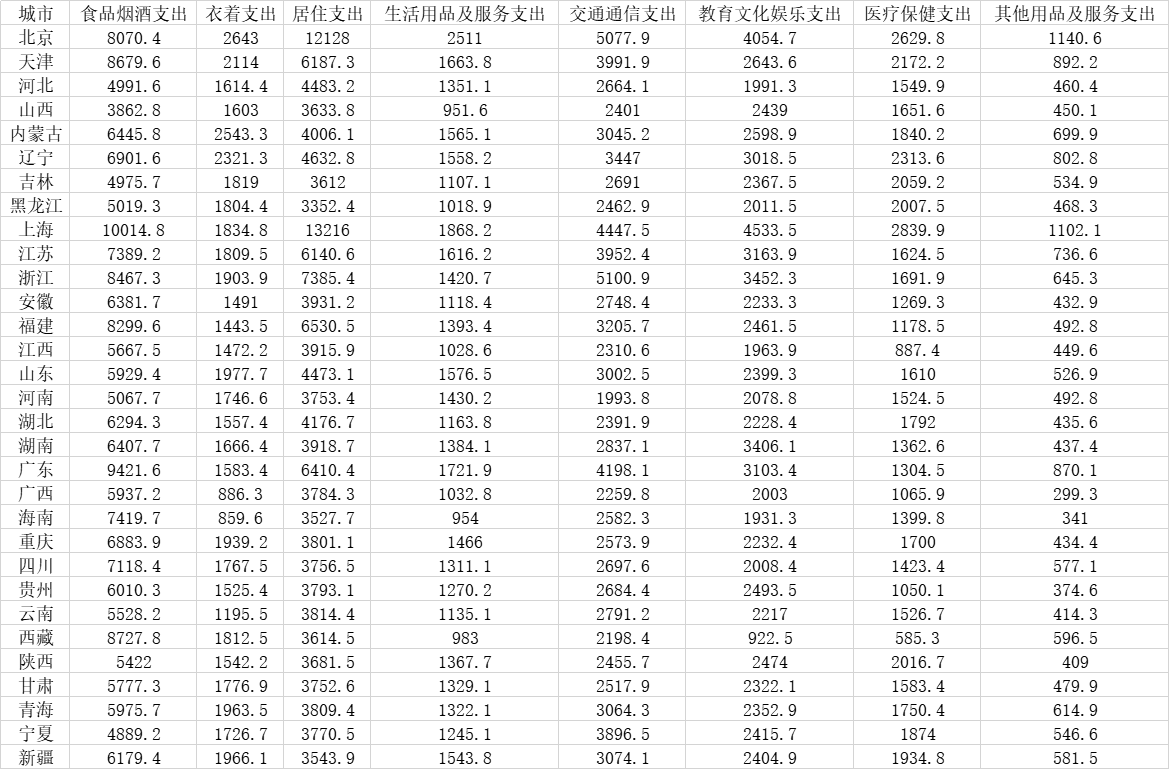数据来源于中国统计年鉴。

## 1.导入库

``````# 数据处理
import pandas as pd
import numpy as np

# 绘图
import seaborn as sns
import matplotlib.pyplot as plt
# 因子分析
from factor_analyzer import FactorAnalyzer``````

# 2.读取数据

``````df = pd.read_csv("D:\桌面\demo.csv",encoding='gbk')
df``````

输出：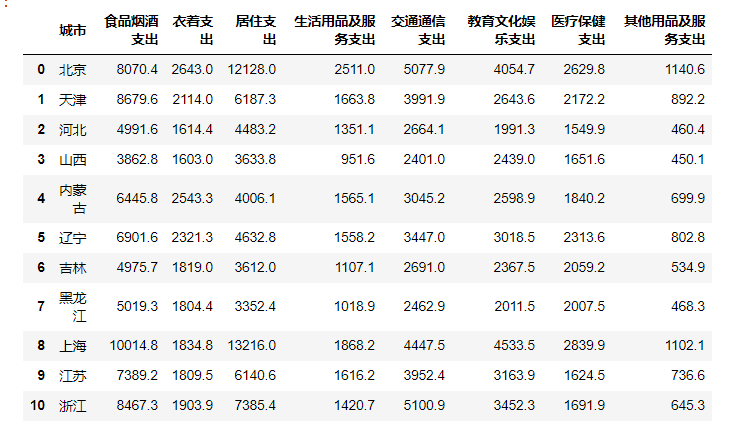如果不想要城市那一列的话，可以在读取的时候就删除，也可以后面再删

比如，读取时删除

``````df = pd.read_csv("D:\桌面\demo.csv", index_col=0,encoding='gbk').reset_index(drop=True)
df``````

返回：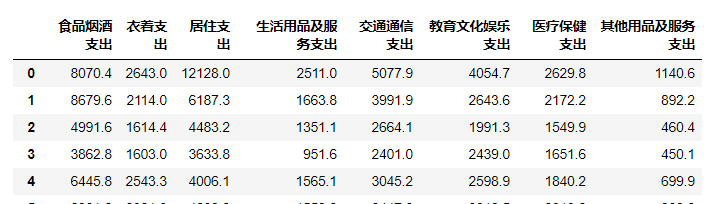然后我们查询一下，数据的缺失值情况：

``df.isnull().sum()``

返回：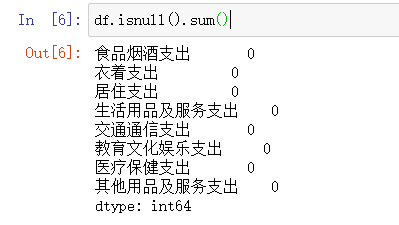然后，我们可以针对的，对数据进行一次处理：

比如删除无效字段的那一列

``````#  去掉无效字段
df.drop(["变量名1","变量名2","变量名3"],axis=1,inplace=True)``````

或者，删除空值

``````# 去掉空值
df.dropna(inplace=True)``````

## 3.充分性检测

在进行因子分析之前，需要先进行充分性检测，主要是检验相关特征阵中各个变量间的相关性，是否为单位矩阵，也就是检验各个变量是否各自独立。

### 3.1 Bartlett's球状检验

检验总体变量的相关矩阵是否是单位阵（相关系数矩阵对角线的所有元素均为1,所有非对角线上的元素均为零）；即检验各个变量是否各自独立。

如果不是单位矩阵，说明原变量之间存在相关性，可以进行因子分子；反之，原变量之间不存在相关性，数据不适合进行主成分分析

``````from factor_analyzer.factor_analyzer import calculate_bartlett_sphericity

chi_square_value, p_value = calculate_bartlett_sphericity(df)
chi_square_value, p_value``````

返回：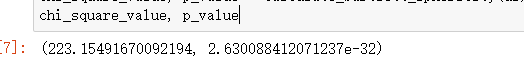### 3.2 KMO检验

检查变量间的相关性和偏相关性，取值在0-1之间；KOM统计量越接近1，变量间的相关性越强，偏相关性越弱，因子分析的效果越好。

通常取值从0.6开始进行因子分析

``````#KMO检验
from factor_analyzer.factor_analyzer import calculate_kmo
kmo_all,kmo_model=calculate_kmo(df)
kmo_model``````

返回：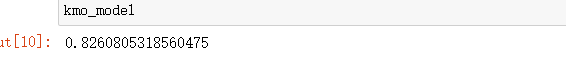通过结果可以看到KMO大于0.6，也说明变量之间存在相关性，可以进行分析。

## 4.选择因子个数

方法：计算相关矩阵的特征值，进行降序排列

### 4.1 特征值和特征向量

``````faa = FactorAnalyzer(25,rotation=None)
faa.fit(df)

# 得到特征值ev、特征向量v
ev,v=faa.get_eigenvalues()
print(ev,v)``````

返回：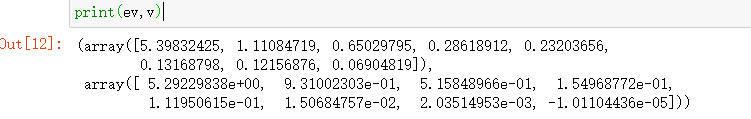### 4.2 可视化展示

将特征值和因子个数的变化绘制成图形：

`````` # 同样的数据绘制散点图和折线图
plt.scatter(range(1, df.shape + 1), ev)
plt.plot(range(1, df.shape + 1), ev)

# 显示图的标题和xy轴的名字
# 最好使用英文，中文可能乱码
plt.title("Scree Plot")
plt.xlabel("Factors")
plt.ylabel("Eigenvalue")

plt.grid()  # 显示网格
plt.show()  # 显示图形``````

返回：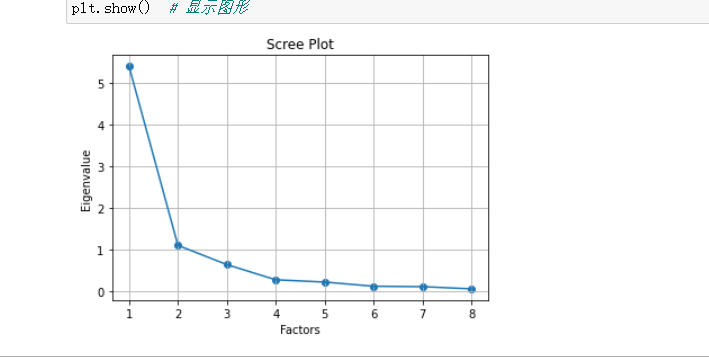从上面的图形中，我们明确地看到：选择2或3个因子就可以了

### 4.3 可视化中显示中文不报错

只需要在画图前，再导入一个库即可，见代码

``````import matplotlib as mpl

mpl.rcParams['font.sans-serif'] = ['SimHei']  # 指定默认字体
mpl.rcParams['axes.unicode_minus'] = False  # 解决保存图像是负号'-'显示为方块的问题``````

## 5.因子旋转

### 5.1 建立因子分析模型

在这里选择，最大方差化因子旋转

``````# 选择方式： varimax 方差最大化
# 选择固定因子为 2 个
faa_two = FactorAnalyzer(2,rotation='varimax')
faa_two.fit(df)``````

返回：ratation参数的其他取值情况：

• varimax (orthogonal rotation)

• promax (oblique rotation)

• oblimin (oblique rotation)

• oblimax (orthogonal rotation)

• quartimin (oblique rotation)

• quartimax (orthogonal rotation)

• equamax (orthogonal rotation)

• ### 5.2 查看因子方差-get_communalities()

查看公因子方差

``````# 公因子方差
faa_two.get_communalities()``````

返回：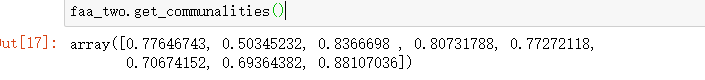查看每个变量的公因子方差数据

``pd.DataFrame(faa_two.get_communalities(),index=df.columns)``

返回：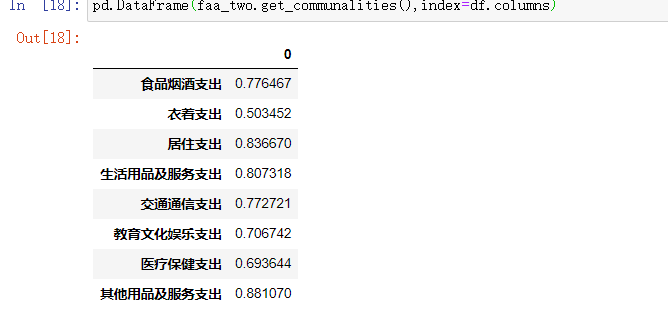### 5.3 查看旋转后的特征值

``faa_two.get_eigenvalues()``

返回：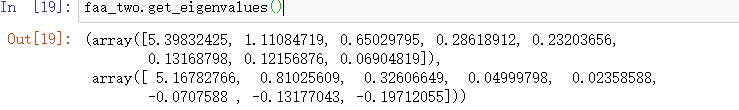``pd.DataFrame(faa_two.get_eigenvalues())``

返回：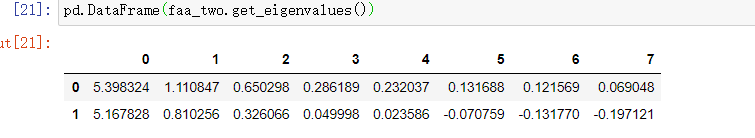### 5.4 查看成分矩阵

查看它们构成的成分矩阵：

``````# 变量个数*因子个数

返回：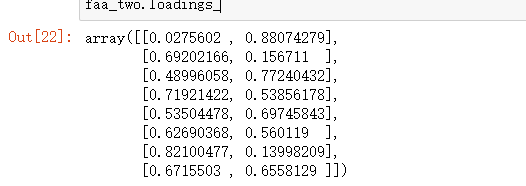如果转成DataFrame格式，index就是我们的变量，columns就是指定的因子factor。转DataFrame格式后的数据：

``pd.DataFrame(faa_two.loadings_,index=df.columns)``

返回：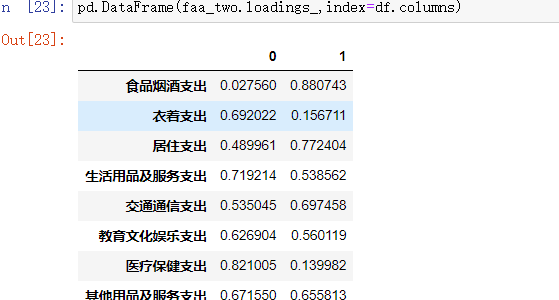### 5.5 查看因子贡献率

通过理论部分的解释，我们发现每个因子都对变量有一定的贡献，存在某个贡献度的值，在这里查看3个和贡献度相关的指标：

• 总方差贡献：variance (numpy array) – The factor variances

• 方差贡献率：proportional_variance (numpy array) – The proportional factor variances

• 累积方差贡献率：cumulative_variances (numpy array) – The cumulative factor variances

•  我们来看一下总方差贡献吧

``faa_two.get_factor_variance()``

返回：## 6.隐藏变量可视化

为了更直观地观察每个隐藏变量和哪些特征的关系比较大，进行可视化展示，为了方便取上面相关系数的绝对值：

``````df1 = pd.DataFrame(np.abs(faa_two.loadings_),index=df.columns)
print(df1)``````

返回：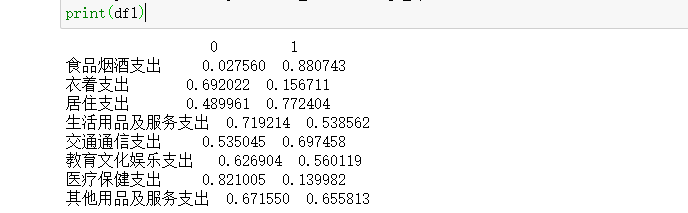然后我们通过热力图将系数矩阵绘制出来：

``````# 绘图

plt.figure(figsize = (14,14))
ax = sns.heatmap(df1, annot=True, cmap="BuPu")

# 设置y轴字体大小
ax.yaxis.set_tick_params(labelsize=15)
plt.title("Factor Analysis", fontsize="xx-large")

# 设置y轴标签
plt.ylabel("Sepal Width", fontsize="xx-large")
# 显示图片
plt.show()

# 保存图片
# plt.savefig("factorAnalysis", dpi=500)``````

返回：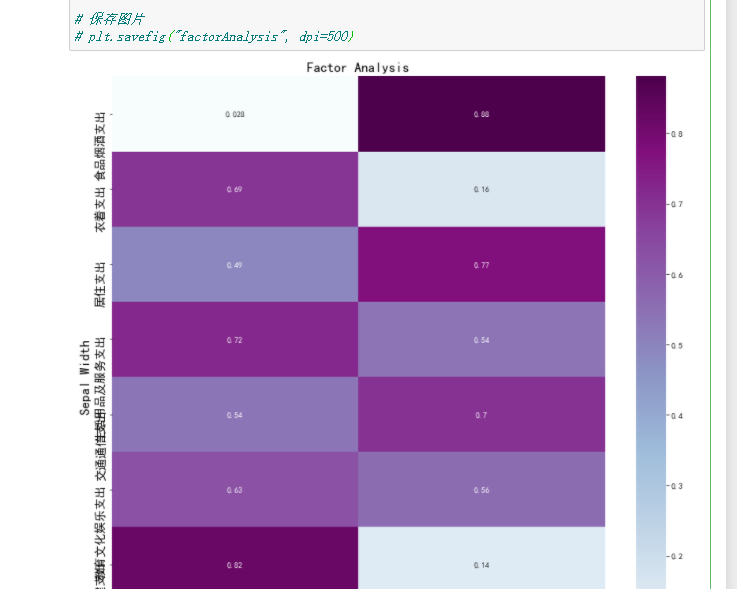## 7.转成新变量

上面我们已经知道了2个因子比较合适，可以将原始数据转成2个新的特征，具体转换方式为：

``faa_two.transform(df)``

返回：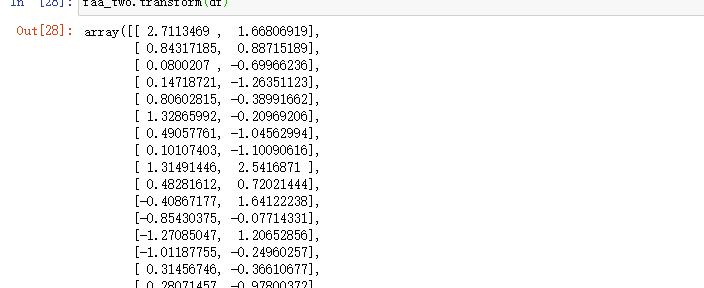转成DataFrame格式后数据展示效果更好：

``````df2 = pd.DataFrame(faa_two.transform(df))
print(df2)``````

返回：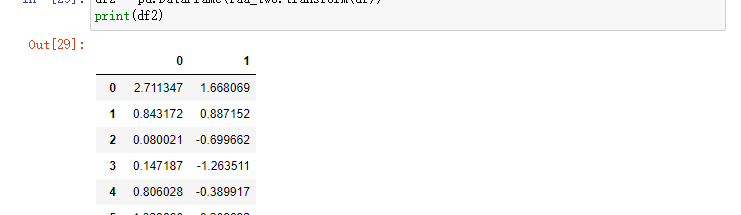# 五·、参考资料

1、Factor Analysis：Factor Analysis with Python — DataSklr

3、`factor_analyzer package`的官网使用手册：factor_analyzer package — factor_analyzer 0.3.1 documentation

4、浅谈主成分分析和因子分析：浅谈主成分分析与因子分析 – 知乎

来源：洋洋菜鸟

物联沃分享整理
物联沃-IOTWORD物联网 » 因子分析——python